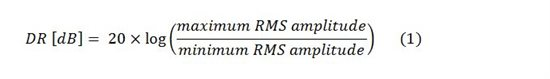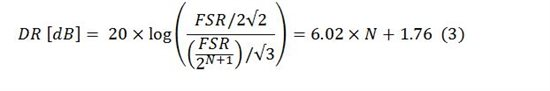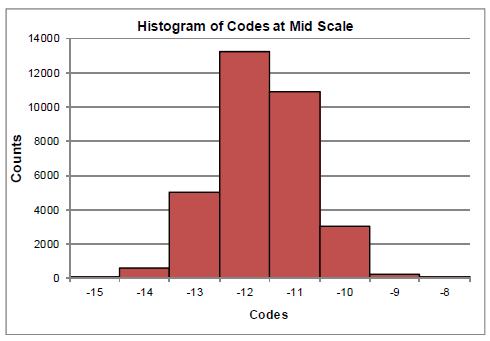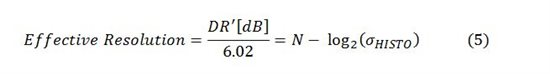# A bone of contention: ENOB or effective resolution?

This technical article was updated on July 23, 2020.As you might know, both ENOB (“Effective Number of Bits”) and effective resolution are parameters that relate to an ADC’s resolution. Understanding how they differ, and deciding which one is more relevant, is a subject of much confusion and frequent debate among ADC users and Applications Engineers alike.

Which one do you think is more important?

The ADC’s number of bits of resolution (N) determines the ADC’s dynamic range (DR), which represents the range of input signal levels that the ADC can measure. Generally specified in [dB] units, DR is defined as:Note that since the RMS amplitude of a signal over a given time window depends on how the signal amplitude varies over that time window an ADC’s DR changes depending on input signal characteristics. For constant DC inputs over its full-scale range (FSR), an ideal N-bit ADC measures maximum and minimum RMS amplitudes of FSR and FSR/2N, respectively. Hence, the ADC’s DR is:Similarly, for sinusoidal inputs with amplitudes varying over the ADC’s FSR, the ideal N-bit ADC measures a maximum RMS amplitude of (FSR/2)/√2. The minimum measurable RMS amplitude for a sinusoidal input is limited by quantization error, which approximates a saw-tooth wave with amplitude half LSB or FSR/2N+1. The RMS amplitude of a saw-tooth waveform of amplitude A is A/√3. Therefore, the DR of an ideal ADC for sinusoidal inputs is:Real ADCs have errors that degrade DR. In fact, depending on input signal characteristics, the ADC output has different types of errors that dominate when the input signal approaches its minimum value.

For constant DC inputs, the ADC's output error is dominated by so-called "transition" noise, which consists of the broadband thermal noise inherent to the ADC, its drivers, power supplies, and so on. If there are no gross linearity (DNL) issues with the ADC, transition noise produces an approximately Gaussian code distribution at the ADC output.Figure 1: Histogram of ADC output codes for a constant DC input

One standard deviation (σHISTO) of this histogram corresponds to the RMS value of the transition noise. For σHISTO > 1 LSB, the ADC’s DC DR decreases to:The decreased resolution or Effective Resolution can be recomputed by combining (2) and (4):Similarly, for time-varying inputs, the ADC's output contains dynamic errors, namely, quantization noise and distortion, in addition to transition noise that degrade DR. The altered DR is commonly known as SINAD, and the recomputed ADC resolution is known as ENOB. Therefore,In summary, a given ADC can have different DRs and resolutions depending on whether the input is an AC or DC signal. Hence, there are separate metrics of ADC resolution that correspond to different input conditions – ENOB for AC inputs, Effective Resolution for DC inputs. Naturally, deciding which is more appropriate depends on your application.

For an in depth look at optimizing the dynamic performance of your High Precision SAR ADC design, check out our webinar on EDN.

• Hello I watch the webinar on EDN , Thank you for your post.

• In SBAS607B, Revised September 2016, the datasheet for the ADS127L01 gives a formula for ENOB that is flatly wrong, overstating ENOB by 1.79 bits.  The definition given in the article above as equation (6) is correct, and agrees with the IEEE standard definition.  The definition given in the data sheet seems to have dropped from the sky, a gift from the technical marketing department to the sales force.  Calling a a daisy a rose doesn't make it one.

What really puzzles me is that the specifications for the ADS127L01 are very good all by themselves, and the substitution of "Effective Resolution" as defined above but calling it ENOB is at best confusing and misleading at worst.